Few Random Questions On Basics

1. What happens to the solar energy which earth receives in such a huge quantity?

We know conversation of energy theorem.  So, is the energy of the earth is constantly rising? A part of energy what we receive gets reflected back to universe, and then what happens to the other part of it?

Under the sun, we feel hot and that confirms that we are receiving the solar energy. In the same way, in night, we feel cold; reason is we are giving back the energy.

(If the energy is transferred from one body to another, than the body which is giving the energy will feel the coldness and others feel the hotness.)

2. Why multiplications of 2 negative numbers yield a positive number?

This is a very basic principle in mathematics which we all know and we are using it all the way from our childhood days.

• How this came and why is it true?

Let’s prove it.

In fact negative numbers are the reflection of the positive numbers (Their magnitude) about the origin in the number line. And while multiplying 2 negative numbers, their magnitude is multiplied first. For 2 negative sign, two times reflection is taken resulting in the product to be a positive number.

3. What might’ve happened if the earth didn’t have gravity?

It’s impossible by the fact that anything which owes mass will have the property of gravity too.

4. Why concentration of air goes on decreasing as the altitude increases?

Atmosphere is present in the earth because of its gravitational power and as altitude increases, force decreases and hence the air concentration will also decrease.

5. What might’ve happened if the earth isn’t tilted to 23.5◦ to its axis of rotation?Google is indisputedbly the best search engine out there on the Internet, however there are a few ways by which you can customize Google and make it even better.

Using OptimizeGoogle, you can get rid of text ads from Google search results, add links from about 10 other search engines, add position counter, product results and more. You can even filter your search results to see dead websites (using WayBack Machine) and remove click tracking so that you can search anonymously. Here is a list of some of the other useful features of this plugin.

1. Use Google suggest (get word suggestion while typing)

2. Add more security by using https wherever necessary

3. Filter spammy websites from search results page

4. Option to remove SideWiki

To install the plugin, just visit the OptimizeGoogle page from your Firefox browser and click on the Download button. Once installed, you can enable or disable the customization options from the Tools -> OptimizeGoogle Options.

After you customize Google to improve the search results, how about adding a feature that provides a way to preview the website in the search results itself? Here is a miracle Firefox extension to this job for us.

2.  SearchPreview:

SearchPreview (formerly GooglePreview) will insert thumbnail view of the webpage into the Google search results page itself so that you can take the guess work out of clicking a link. Just install the plugin, reload Firefox and you will have the SearchPreview at work. You can install this plugin from the following link.

OptimizeGoogle and SearchPreview have made our search results smarter and faster. Now how about safer? Well you have another Firefox plugin to make your search results safer as well. Here we go.

This is a free browser plugin that gives safety advice about websites on the search results page before you actually click on the links. After you install the SiteAdvisor plugin, you will see a small rating icon next to each search result which will alert you about suspecious/risky websites and help you find safer alternatives. These ratings are derived based on various tests conducted by McAfee.

Based on the quality of links, SiteAdvisor may display Green, Yellow, Red or Grey icon next to the search results. Green means that the link is completely safe, Yellow means that there is a minor risk, Red means a mojor risk and Grey means that the site is not yet rated. These results will guide you to Web safety.SEARCH Techniques:

Using Google it is possible to gain access to an email repository containing CV of hundreds of people which were created when applying for their jobs. The documents containing their Address, Phone, DOB, Education, Work experience etc. can be found just in seconds.

intitle:”curriculum vitae” “phone * * *” “address *” “e-mail”

You can gain access to a list of .xls (excel documents) which contain contact details including email addresses of large group of people. To do so type the following search query and hit enter.

filetype:xls inurl:”email.xls”

Also it’s possible to gain access to documents potentially containing information on bank accounts, financial summaries and credit card numbers using the following search query

intitle:index.of finances.xls

2. Using Google to access Free Stuffs

Ever wondered how to get free music or ebooks. Well here is a way to do that. To download free music just enter the following query on google search box and hit enter.
“?intitle:index.of?mp3 akon“

Now you’ll gain access to the whole index of akon album where in you can download the songs of your choice. Instead of eminem you can subtitute the name of your favorite album. To search for the ebooks all you have to do is replace “akon” with your favorite book name. Also replace “mp3″ with “pdf” or “zip” or “rar”.

The “intitle:” syntax helps Google restrict the search results to pages containing that word in the title. For example, “intitle: login password” (without quotes) will return links to those pages that has the word “login” in their title, and the word “password” anywhere in the page.

Similarly, if one has to query for more than one word in the page title then in that case “allintitle:” can be used instead of “intitle” to get the list of pages containing all those words in its title. For example using “intitle: login intitle: password” is same as querying “allintitle: login password”.

The “inurl:” syntax restricts the search results to those URLs containing the search keyword. For example: “inurl: passwd” (without quotes) will return only links to those pages that have “passwd” in the URL.

Similarly, if one has to query for more than one word in an URL then in that case “allinurl:” can be used instead of “inurl” to get the list of URLs containing all those search keywords in it. For example: “allinurl: etc/passwd“ will look for the URLs containing “etc” and “passwd”. The slash (“/”) between the words will be ignored by Google.

Golden Rectangle and Golden Ratio

What is the Golden RatioIn a golden rectangle, it can be divided into a square and a smaller rectangle. The ratio of the width of the small rectangle to the width of the square is the same as that of the width of the square to the entire rectangle. In fact, when you divide a golden rectangle into a square and smaller rectangle, the smaller rectangle is a golden rectangle as well.

Golden ration in NatureThe most common example of the golden ratio is the nautilus shell (see image). As it spirals in on itself, the spirals get smaller and smaller in the same proportion to each other as they do to the whole. You can also see the ratio in things like sunflower petals, and the curvature of fern fronds (see image).

Let’s look at the golden ratio, a little bit. You can verify these observations algebraically.

1. What is one over the golden ratio? 1 / 1.61803398875 . . .=0.61803398875 . . . Does that answer look familiar? It is one less than the golden ratio.
2. What is the golden ratio squared? (1.61803398875 . . .)2=2.61803398875 . . . Does that answer look familiar? It is one more than the golden ratio.
3. I was trying to investigate chaos, and I drew the graph of y=x2-1. This parabola intersects the graph of the line y=x in two points. What are the coordinates of those two points? The points are (-0.61803398875 . . .,-0.61803398875 . . .) and (1.61803398875 . . .,1.61803398875 . . .). All four of those numbers should look familiar by now. Of course, the x and y coordinates are identical, for both points, as the points are on the line y=x.

Here is an infinite series which evaluates to the golden ratio:

ø=1+1/1·1-1/1·2+1/2·3-1/3·5+1/5·8-1/8·13+…

Notice the alternating signs. Each denominator is the product of two consecutive Fibonacci numbers. Where did I get this series? I deduced it from the fact that the ratio of consecutive Fibonacci numbers approaches the golden ratio, as the numbers get larger. The sequence of ratios of consecutive Fibonacci numbers is 1/1, 2/1, 3/2, 5/3, 8/5, 13/8,… We get the second element of this sequence with this series: 1+1/1·1. The third element is 1+1/1·1-1/1·2. The fourth is 1+1/1·1-1/1·2+1/2·3, and so on. This series was known long before I discovered it.The golden ratio can be represented as the simplest continued fraction (as shown on the left). This fraction is 1+1/a where a is 1+1/a. You probably evaluate such a continued fraction from the right side, and work toward the left, except that it has infinitely many terms. We can get a sequence from this by starting at the left, and evaluating only part of the continued fraction: 1, 2/1, 3/2, 5/3, 8/5, 13/8… These are the ratios of consecutive Fibonacci numbers.The golden ratio can be represented as a repeated square root, as in the diagram at the right. Evaluate this from right to left, too.

Magic of special Numbers.

153

We begin this page of special numbers with one of my all-time favorites: 153. The reason I like it so much, I guess, is that it was the first one I learned about many years ago from a number category called narcissistic. Its unique quality, and what qualifies it as narcissistic, can be stated in these words: it is equal to the sum of the cubes of its digits. Observe:

153 = 13 + 53 + 33

= 1 + 125 + 27

= 153

Now, how’d you like that? Clever, huh! Well, there are only three other numbers that share this same quality. They are also three-place numbers. Can you find them?

Now, here are two more reasons why 153 is special.

If you add up all the whole numbers from 1 to 17, you get 153. This can be expressed mathematically in this way:

153 = 1 + 2 + 3 + 4 + … + 16 + 17

This is the same as saying that 153 is the 17th triangular number.

The other reason that 153 is interesting uses the concept of factorial. Recall that n! means “n(n-1)(n-2)…2×1.” So look at this expression:

1! + 2! + 3! + 4! + 5!

What do you think it equals? Right! 153.

extra curious fact about 153 can be shown…

sqrt(153 – SoD(153)) = 15 – 3.

where SoD(n) means the “Sum of the Digits of n”.

1634

Continuing with the theme of 153, with but one slight and rather important change, I will show why 1634 is rather special too. The change this time is that we will use the fourth powers of the digits. This gives this equation:

1634 = 14 + 64 + 34 + 44

= 1 + 1296 + 81 + 256

= 1634

Will numbers ever cease to amaze me? I hope not. There are two more cases like this one. Again we challenge you to find them.

Mathematicians call such numbers as those we’ve just discussed above as PDI’s (Perfect Digital Invariants). A PDI is a number that can be expressed as some combination of its digits and various operations, powers, or whatnot. The two numbers discussed so far used the cubes and 4th powers of the digits. Numbers that use higher powers also exist; some examples include:

54,748 and fifth powers,

548,834 and sixth powers,

1,741,725 and seventh powers.

Do you care to verify these cases, or do you trust me by now? 😉

1729

This special number, which involves cubes in a different way than described above, has a special story to go along with it. Some time ago in the early part of this century, a famous young mathematician from India was visiting the noted English mathematician Hardy in London. Ramanujan, the Indian, had become sick and Hardy had gone to see him. Hardy later wrote of the incident, saying: “I had ridden in a taxi-cab No. 1729, and remarked to my guest that the number seemed to me a rather dull one, and that I hoped it was not an unfavorable omen. ‘No’, he remarked, ‘it is a very interesting number; it is the smallest number expressable as the sum of two cubes in two different ways.'”

Here is what Ramanujan meant:

1729 = 13 + 123 = 93 + 103

= 1 + 1728 = 729 + 1000

By way of asking an easier question so that you may better appreciate this concept:

What is the smallest number that is expressable as

the sum of two distinct squares in two different ways?

Three final notes about 1729 that I think are equally interesting:

1st: If you factor it into primes, you get

1729 = 7 × 13 × 19

and those primes form an arithmetical sequence with a common difference of 6, making them “special” primes .

2nd: Then by combining 7 and 13 into one factor and rearranging the order, we have

1729 = 19 × 91

That’s sort of a palindromic arrangement of the digits, right?

3rd: If we note that the sum of the digits of 1729 is 19, then this is sufficient to declare that “1729 is a Niven number!”

Wow! 1729 is some fantastic number, for sure.

635,318,657

I can hear you saying on this one: “Now how can that monster of a number be special?” It’s really quite simple if I tell you to use Ramanujan’s idea just explained above. Believe it or not, but it is the smallest number that is express-able as the sum of two 4th powers in two different ways.

If I present this in symbols of algebra, it would look like this:

635,318,657 = A4 + B4 = C4 + D4

where A, B, C, and D are distinct whole numbers.

Of course, you’d like to know what those letters stand for, wouldn’t you? To give them out to you directly is not the way of “Trotter Math”; but I will give you some clues, okay?

Clue #1: One pair of numbers are consecutive, in the 130-140 range.

Clue #2: One number of the second pair is 59.

Now you can find the numbers.

An historical fact about this number is that it was discovered by the brilliant Swiss mathematician Leonhard Euler in the 18th century.

145

This is a very special number, even though at first glance there seems to be nothing unique about it. In fact, it’s another narcissistic number but in a way different than what was discussed earlier. To understand why, we need to know the meaning of a special mathematical symbol: “!” That’s it; the ordinary exclamation point.

But in math, it is called the factorial symbol. It is used in this way:

5! = 5 × 4 × 3 × 2 × 1 = 120.

We read that as “5-factorial”. So it means nothing more nor less than the product of the given whole number with all the smaller ones down to 1.

So what does this have to do with 145, you ask? Well, just observe this neat little statement:

145 = 1! + 4! + 5!

= 1 + 24 + 120

= 145

See? We are back to 145 again. Now that’s special, wouldn’t you agree?

24

In addition to being two dozen and 4! (4-factorial), the number 24 has another interesting characteristic. If you select any prime number, greater than 3, square it, then diminish that by 1, then 24 is always a divisor (factor) of the result.

Here is an example:

1. Choose the prime 17.

2. Its square is 289.

3. Subtracting 1 gives 288.

4. Then 288 divided by 24 gives exactly 12.

See? It works. I kid you not. Now you might wish to test a few more primes of your own choosing, just so you believe it more firmly. (Then later you can amaze your friends with your new found knowledge.)

A good activity here for students of Algebra is to PROVE that it always works. Just using many examples is not considered as proof in mathematics. (However, if many examples do work out, it is, I suppose, an indication that something “might” be true.)

142857

Probably you recognize this number; it’s the 6-digit period of the rational number 1/7.

1

— = 0.142857142857142857…

7

It is a favorite of math buffs because it has some unusual characteristics when multiplied by other numbers. But one that seems to be overlooked in most books is the fact that the missing digits are 0, 3, 6, and 9, which are the multiples of 3. (This is an observation that becomes more important when one investigates the periods of other fractions.)

But back to our multiplying idea… Note these products.

142857 × 2 = 285714

142857 × 3 = 428571

142857 × 4 = 571428

142857 × 5 = 714285

142857 × 6 = 857142

When you multiply by 7, you get a surprise. Do it!

Here is another trick to show how special the number is. Separate it into two halves, and then add.

142857

142 + 857 = 999

Next split it into thirds before adding.

142857

14 + 28 + 57 = 99

Finally, let’s square the number before splitting and adding.

1428572 = 20,408,122,449

What did you find, my friend?

92

The year 1992 has been gone for some time now, but the short form of the year ’92 is a number that produces a lot of 9’s (remember what happened to the previous entry just above?). (I found this idea in a book titled “Every Number Is Special” by Boyd Henry.) It is done in this unusual manner:

Multiply 92 by 8, then that product by 8, then that product by 8, etc. List the products one under the other, shifting the digits two places to the right as shown below. Continue indefinitely. Add. The sum converges to a string of 9s.

92

736

5888

47104

376832

3014656

24117248

9999999…

Not to let the full year number be left out, WTM takes pleasure in pointing out that 1992 is sorta nice by itself. Look:

1992 = 8 × 3 × 83

WTM now hopes that you will take a new interest in the world of numbers and try to uncover their inner personalities. They’re a little like we people, each unique in some way, yet at the same time, sharing common properties with others.

Thanks to http://www.trottermath.net

Can we see sound and hear electromagnetic waves??!!

Suddenly this question came to mind. I was thinking, since both are waves, both have frequency and wavelength, But why can’t we see sound waves and hear to light rays?!! 😛 😀

Later i came to know reasonably good the answer for second one. No We can’t hear light waves. Sound waves are the waves which propagate through the medium through vibrations. Where as Electromagnetic waves doesn’t make any particle to vibrate or shake…! And Hence We Can’t.

Is it possible to have a Light Absorbing Torch?

In this universe, we believe everything is made in pair. Or we think so.. Main reason for this is our assumption, that is Nature loves symmetry. From Nearly a month back (or more than that) im having a query within myself. Do we have in this world or Can we make something which performs reverse action of a torch..?  That is absorbing a portion of light where we focus on..? (For my convenience let me call it as a Light Absorbing Torch)At least can we make the brightness of the region where we focus the Light absorbing torch, a little low compared to earlier?!

An article Of Surein Aziz

Often when reading a good maths book, the author will get to the end of an explanation of a particularly complicated proof, theorem, or idea, and mention the “beauty” of the maths involved. I always wonder what, exactly, this means. Did I miss a particularly neat diagram? Or, as seems to be the case, is mathematical beauty something buried deep: something that, perhaps, I need a PhD to get to grips with?

I used to think that it was the latter — maybe one day, after years of studying maths at its highest level, I’d suddenly gain a glimpse of some incomprehensibly deep truth and realise the incredible beauty of things which now seem boring and trivial.

Maths can be like a dense jungle — it’s hard to penetrate but you never know whom you might might.

But actually, I think you can get a glimpse of what mathematicians mean by beauty without too much effort at all. That’s what I’m going to try and convince you of in the rest of this article. Mathematics can be a bit like a dense, never-ending jungle. It can feel like you’re hacking away and away at it and never getting anywhere, but if you stop and look around yourself, every once in a while you see incredible, exotic plants and animals to marvel at — and ever so often you find large new swathes of jungle to explore.

The particular thing that I want to introduce you to, that I think is so beautiful, is something that was mentioned in passing on a television programme I was watching. I hardly knew what it meant, and I certainly had no idea how it came about, but I knew I had to find out more.

I am talking about Euler’s identityNow you probably think I’m crazy. What’s beautiful about that? Well, I ought to warn you, I’m not alone — Mathematical Intelligencer readers voted the identity the “most beautiful theorem in mathematics”. The physicist Richard Feynman called the formula it is derived from “one of the most remarkable, almost astounding, formulas in all of mathematics”.

But what is so special about it? Well, first I ought to explain what the symbols actually mean.

You’re probably familiar with, it’s the ratio between a circle’s circumference and its diameter. The numberis also a constant, and you may be vaguely familiar with it as the base of the natural logarithm. To 20 decimal places,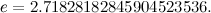Bothandare irrational numbers – they have an infinite number of decimal places and you can’t write them down as one integer divided by another.

Probably the strangest of these three numbers is. It is the square root of -1, that is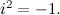It’s called an imaginary number, and you can’t find it anywhere along the normal number line, as none of the ordinary real numbers give a negative number when squared.

Are you starting to get an idea of the beauty of Euler’s identity? If you take the constantto the power of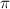multiplied by, and then take away 1, you get to 0. Isn’t it a little odd how three very strange numbers which are not connected in any evident way combine to give such a normal and familiar result?Euler’s identity is named after Leonhard Euler, one of the most prolific mathematicians of all times.

So, why does this happen? You might think that it is down to some really complex idea — how do we even take a number to the power of? Well, actually, it isn’t too difficult to see how Euler’s identity comes about – that is one thing that makes the identity so wonderful! But first you have to see Euler’s formula, which leads to his beautiful identity, in full generality:Doesn’t look quite as nice and neat now, does it? But don’t be put off. To understand how this formula comes about, we need something called Taylor series. These are just a way of expressing functions such as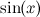or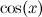as infinite sums. They were discovered by the mathematician Brook Taylor (who was also part of the committee which adjudicated the argument between Isaac Newton and Gottfried Leibniz about who first invented the calculus).

The Taylor series for the function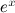is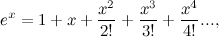wheredenotes the productYou can verify this Taylor series using a calculator: choose a numberand see what value the calculator gives you for. Now use the calculator to work out the value of the sum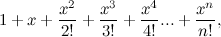for as many terms as you like, that is for a number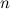as high as you like. You will find that the result very nearly equals the result you got forand the more terms you add to the sum, the closer the two results become. At some point the two results will be the same on your calculator, as their difference becomes too small for the calculator to detect. In “reality”, the two results are equal when you have added an infinite number of terms to your sum.

The Taylor series for the other two functions appearing in Euler’s formular are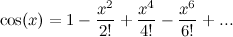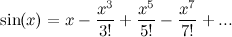Again you can check this using your calculator, bearing in mind that the angle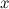is measured in radians, rather than degrees.

Now let’s multiply the variablein the Taylor series forby the number. We getBut certain powers ofcan be simplified – for example,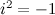by definition, and so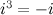and, and so on. So we can simplify the above toWe can gather the terms involvingtogether to give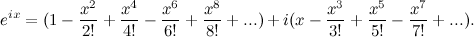Now notice that these two series are the same as the series forandfrom earlier, so we can substitute these in to get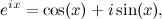which is Euler’s formula!

All we have to do now is substitute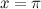. Sinceandwe get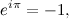soSo you see, after a sequence of fairly complex mathematics we arrive back where we started — at the (seemingly) simple numbers 1 and 0. That is what I think is so beautiful about this identity: it links very strange numbers with very ordinary and fundamental ones. Seeing why it works feels a bit like treading a little-known path through the mathematical jungle to reach a secret destination somewhere in the thick undergrowth.